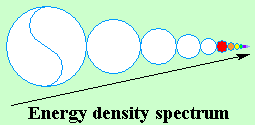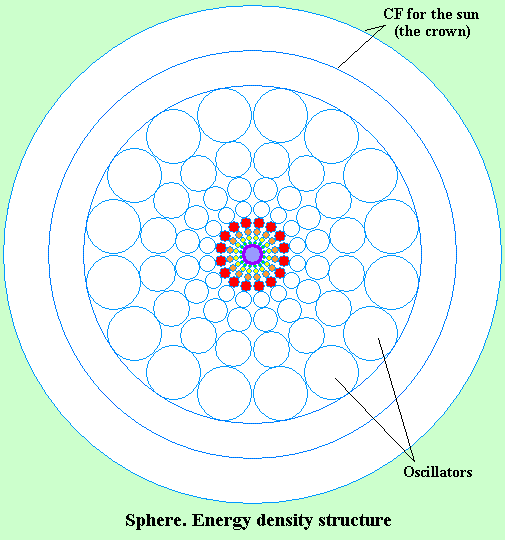The specter of electromagnetic oscillations

Sir Isaac Newton did a brilliant affirmation! "I do not invent hypotheses"
According to the dictionary, hypothesis is an assumption, enounced based on known facts, related to certain (connections amongst) phenomena which cannot be noticed directly or related to the essence of phenomena, to the cause or internal mechanism which produces them.
Newton's affirmation means as well that hypothesis may be "imposed", "invented"
An a priori imposed hypothesis is the electron and therefore, all the assembly of hypotheses which followed, planetary atom, spin, proton, neutron and all the particles which intermediate the reports amongst them.
(Electron generated "catastrophes" and "uncertainties")
The specter of electromagnetic oscillations has four important sizes:
Frequency, wavelength, propagation speed and energy.
The constant "c" shall result out of the product of frequency and wave length
Energy is a property of curve polarized space, of the existence vector.
Attraction and rejection forces are current and voltage in the curve polarized space!
Current and voltage are properties of the space, of the existence vector!!!
Oscillation represents variations of elementary and centripetal forces in toroidal space.
Elementary force varies inversely proportionally to the volume of the torus, and centripetal force is a sum of elementary forces and grows directly proportional to the volume of the torus.
There results that the energy of oscillation equals the square of elementary force!
The energy of the oscillation is a constant of the space, of the existence vector!
In the double-torus oscillator, the diminution of the volume of a torus, orthogonally induces the amplification of the other torus (Faraday phenomena).
Oscillation, variation of the volume are induced, energy remaining constant.
At least apparently, this conclusion contradicts the reasoning according to which the energy of a photon is the product between the frequency of oscillation and Planck's constant, that is E = h f.
(the constant is tightly connected to the operation of the phantom electron)
Based on this formula, the frequency specter expresses as well an energy spectrum.
Actually, it expresses energy density.
We make with such specter a sphere with the maximum frequency to the centre.
We obtained a hierarchy of energy density, a corpus like the structure of the sun..
Energy density and frequency grow towards the centre of the sphere.<
>